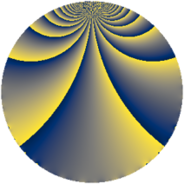# Properties

 Label 712.1.sLevel $712$ Weight $1$ Character orbit 712.s Rep. character $\chi_{712}(67,\cdot)$ Character field $\Q(\zeta_{22})$ Dimension $10$ Newform subspaces $1$ Sturm bound $90$ Trace bound $0$

# Related objects

## Defining parameters

 Level: $$N$$ $$=$$ $$712 = 2^{3} \cdot 89$$ Weight: $$k$$ $$=$$ $$1$$ Character orbit: $$[\chi]$$ $$=$$ 712.s (of order $$22$$ and degree $$10$$) Character conductor: $$\operatorname{cond}(\chi)$$ $$=$$ $$712$$ Character field: $$\Q(\zeta_{22})$$ Newform subspaces: $$1$$ Sturm bound: $$90$$ Trace bound: $$0$$

## Dimensions

The following table gives the dimensions of various subspaces of $$M_{1}(712, [\chi])$$.

Total New Old
Modular forms 30 30 0
Cusp forms 10 10 0
Eisenstein series 20 20 0

The following table gives the dimensions of subspaces with specified projective image type.

$$D_n$$ $$A_4$$ $$S_4$$ $$A_5$$
Dimension 10 0 0 0

## Trace form

 $$10q - q^{2} - 2q^{3} - q^{4} - 2q^{6} - q^{8} - 3q^{9} + O(q^{10})$$ $$10q - q^{2} - 2q^{3} - q^{4} - 2q^{6} - q^{8} - 3q^{9} + 9q^{11} - 2q^{12} - q^{16} + 9q^{17} - 3q^{18} - 2q^{19} - 2q^{22} - 2q^{24} - q^{25} + 7q^{27} - q^{32} - 4q^{33} - 2q^{34} - 3q^{36} + 9q^{38} - 2q^{41} - 2q^{43} - 2q^{44} - 2q^{48} - q^{49} - q^{50} - 4q^{51} + 7q^{54} - 4q^{57} - 2q^{59} - q^{64} - 4q^{66} - 2q^{67} + 9q^{68} + 8q^{72} - 2q^{73} - 2q^{75} - 2q^{76} - 5q^{81} - 2q^{82} - 2q^{83} - 2q^{86} - 2q^{88} - q^{89} - 2q^{96} - 2q^{97} - q^{98} + 5q^{99} + O(q^{100})$$

## Decomposition of $$S_{1}^{\mathrm{new}}(712, [\chi])$$ into newform subspaces

Label Dim. $$A$$ Field Image CM RM Traces $q$-expansion
$$a_2$$ $$a_3$$ $$a_5$$ $$a_7$$
712.1.s.a $$10$$ $$0.355$$ $$\Q(\zeta_{22})$$ $$D_{11}$$ $$\Q(\sqrt{-2})$$ None $$-1$$ $$-2$$ $$0$$ $$0$$ $$q-\zeta_{22}^{5}q^{2}+(\zeta_{22}^{4}-\zeta_{22}^{9})q^{3}+\zeta_{22}^{10}q^{4}+\cdots$$